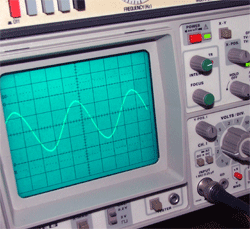# LCR Series Circuits

• Module 9.0 Introduction
•   • Introduction to LCR Series Circuits.
• Section 9.1 LCR Series Circuit Action.
• Recognise LCR Series circuits and describe their action using phasor diagrams and appropriate equations:
•   • Below Resonance
•   • Above Resonance
•   • At Resonance
• Section 9.2 Series resonance.
• Series Resonance
•   • Describe LCR Series Circuits at resonance.
•   • Describe the conditions for series resonance.
•   • Carry out calculations on LCR series circuits, involving reactance, impedance, voltages and current.
• Section 9.3 Voltage Magnification.
• Voltage Magnification.
•   • Describe voltage magnification in LCR Series Circuits
•   • Calculate Voltage Magnification using appropriate formulae.
• Section 9.4 LCR Series Quiz.
•   • LCR Series Circuits Quiz.

## Amazing LCR Circuits.

This module introduces some of the most useful and most amazing circuits in electronics. They can be as simple as two or three components connected in series, but in their operation they can perform many complex tasks and are used perhaps, in more circuit applications than any other circuit arrangement.Connecting an inductor, a capacitor and perhaps a resistor, either in series or in parallel, makes some surprising things happen. Previous modules in this series have examined capacitors and inductors in isolation, and combined with resistors. These have created useful circuits such as filters, differentiators and integrators. Now module 9 looks at what happens when inductors and capacitors are combined in a single circuit network.

Capacitors and inductors act in different (and often opposite) ways in AC circuits. This module is about combining the properties of reactance and impedance of capacitors and inductors with varying frequency to produce amazing effects.

A circuit containing L,C and R at a certain frequency can make L and C (or at least their electrical effects) completely disappear! The LCR circuit can appear to be just a capacitor, just an inductor, or solely a resistor! Not only that, the series LCR circuit can magnify voltage, so the voltages across individual components within the circuit, can actually be much larger than the external voltage supplying the circuit. LCR circuits can also dramatically change their impedance to offer more or less opposition to current at different frequencies. All these effects can be used separately or together to make the wide range of electronic devices that use AC.